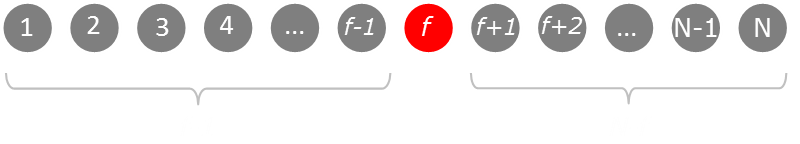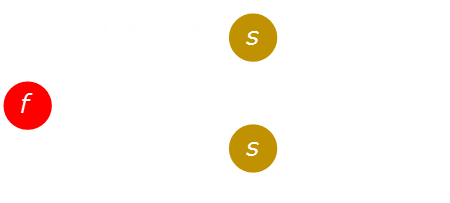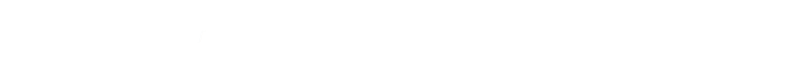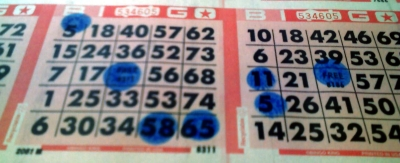﻿ Two Bingo Ball Puzzle# Two Ball Bingo PuzzleImage: Digby Fire Dept There are 75 balls in an American bingo game (according to the internet). From a fully shuffled set of bingo balls, you draw one number. Without replacing this ball, you draw a second number. If you win a prize equal to the value of the highest number you draw, what is your expected return? (Meaning: if you were able to play the game an infinite number of times, what would be your average winnings?)

What is the expected value if you draw two numbers and get to keep the highest?

### Solution

Let's break out a little math. Rather than restricting ourselves to 75 balls, let's determine the generic solution. Let's imagine there are N balls in the game (where N≥2). Without loss of generality, let's imagine we draw our first ball. We'll call the value of the first ball f. Below we can see a graphic representation of this:After the first ball is drawn it splits the remaining balls into two groups (either of which could be zero in length if the ball selected is at one of the extreme ends). The first group is the group of balls that is less than the first selected ball. There are f-1 numbers in this group. The second group contains the balls that are greater than the first selected ball. There are N-f balls in this second group.

It is not possible to draw a number equal to the first drawn number because each number is distinct, and we do no replace.It's now time to draw the second ball. The second ball must be drawn from one of the two groups described above. There are now N-1 balls to select from so it is very easy to calculcate the probability that the second ball will be picked from each of these groups.

The expected value is the sum of the probabilities of each branch multiplied by the expected value of that branch. Explicitly, it's the probability that the second number is lower multiplied by the score obtained on that branch plus the probabiloity the second number is higher multiplied by the score obtained on that branch.If the second number is lower we take the score of the first number drawn, which we know is f (Because we keep the higher of the two balls). If the second number is higher we take the expected value of all the possible higher values, which the average of all the possible numbers that is in the higher group.

This gives us the expected value for any value of fFinally we need to find the expected value for all possible values of f. We can do this by calculating the average over all the possible values for what f can be in the range 1 — NA little bit of rearrangement and then we can calculate the sums over the range 1 — N. What is interesting (and a little surprising?) is that the expected value contribution from both sides, after we have caculated the arithmetic sums, is the same!This gives a very neat a simple formula. Plugging in the value N=75, we find out that the expected value is 50⅔With 75 numbers, the expected value, after two draws and keeping the highest, is 50⅔.

You can find a complete list of all the articles here.Click here to receive email alerts on new articles.